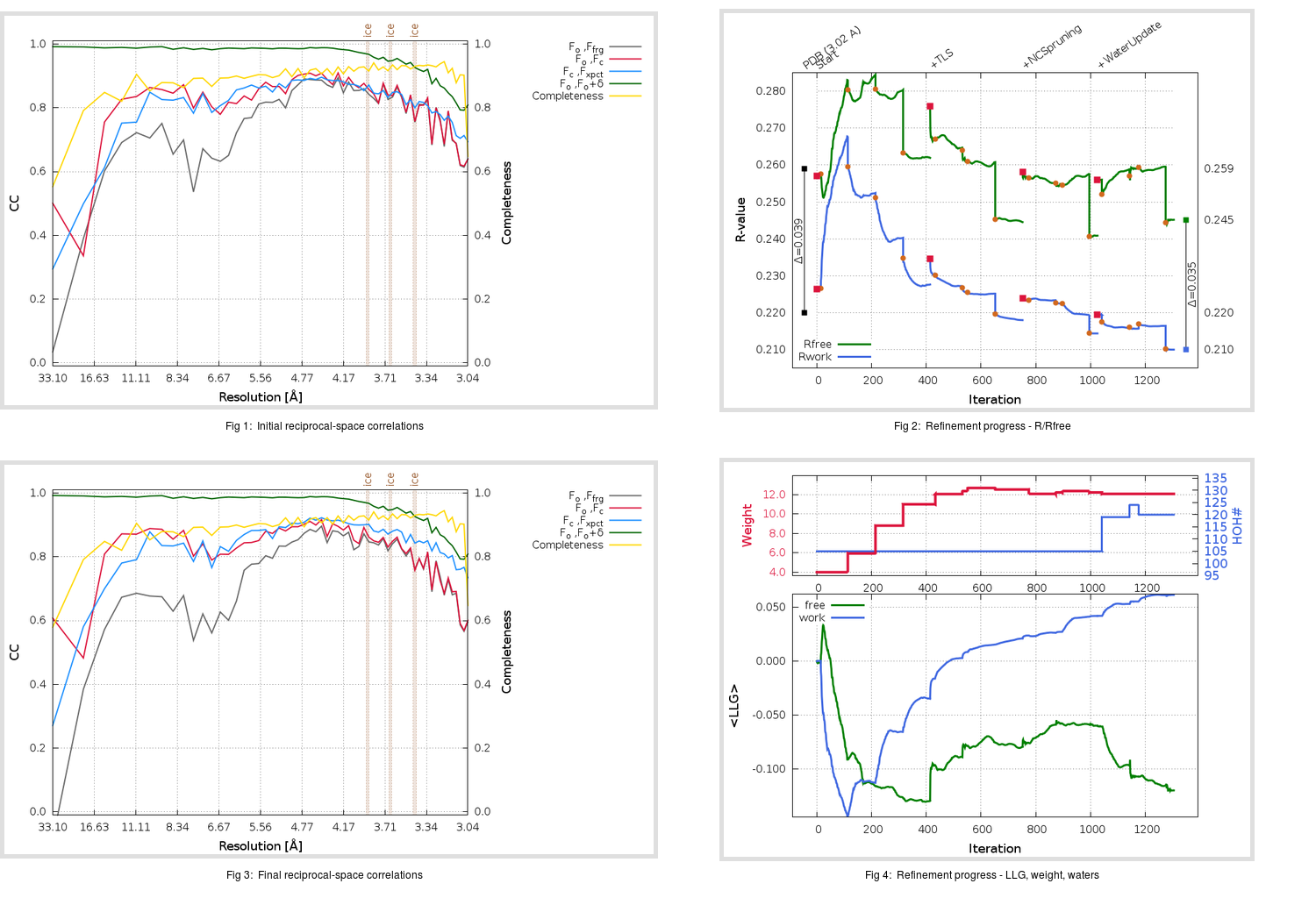Content:

```    Diffraction limits & principal axes of ellipsoid fitted to diffraction cut-off surface:
2.920         1.0000   0.0000   0.0000       a*
3.092         0.0000   1.0000   0.0000       b*
2.976         0.0000   0.0000   1.0000       c*
```

## Deposited

` `
 Date deposited Date data collection Resolution R, Rfree 20150508 20140610 3.02 0.2170 0.2590

Molprobity (CCP4 7.0 version) summary:

```Ramachandran outliers =   0.24 %
favored =  96.60 %
Rotamer outliers      =   5.53 %
C-beta deviations     =     0
Clashscore            =   6.18
RMS(bonds)            =   0.0032
RMS(angles)           =   0.82
MolProbity score      =   2.12
Resolution            =   3.02
R-work                =   0.2170
R-free                =   0.2590
```

```Number of waters      =   105

<B> (all atoms) =   61.26 ( sd =   31.02 ) for       3388 non-hydrogen atoms
<B>   (protein) =   60.09 ( sd =   29.29 ) for       3222 non-hydrogen atoms
<B>     (water) =   48.30 ( sd =   12.56 ) for        105 non-hydrogen atoms
<B>    (others) =  145.49 ( sd =   20.11 ) for         61 non-hydrogen atoms

B min/max       (all non-hydrogen atoms) =   28.48 /  222.35
B min/max   (protein non-hydrogen atoms) =   28.48 /  222.35
B min/max     (water non-hydrogen atoms) =   31.85 /   86.30
B min/max     (other non-hydrogen atoms) =   99.62 /  175.10
```

## BUSTER (re-)refinement

` `

Molprobity (CCP4 7.0 version) summary:

```Ramachandran outliers =   0.24 %
favored =  94.66 %
Rotamer outliers      =   9.74 %
C-beta deviations     =     2
Clashscore            =   6.18
RMS(bonds)            =   0.0120
RMS(angles)           =   1.63
MolProbity score      =   2.46
Resolution            =   3.02
R-work                =   0.2100
R-free                =   0.2452
```

```Number of waters      =   120

<B> (all atoms) =   61.45 ( sd =   23.66 ) for       3403 non-hydrogen atoms
<B>   (protein) =   61.21 ( sd =   23.27 ) for       3222 non-hydrogen atoms
<B>     (water) =   48.77 ( sd =   19.93 ) for        120 non-hydrogen atoms
<B>    (others) =   99.39 ( sd =    9.63 ) for         61 non-hydrogen atoms

B min/max       (all non-hydrogen atoms) =   17.64 /  171.73
B min/max   (protein non-hydrogen atoms) =   29.74 /  171.73
B min/max     (water non-hydrogen atoms) =   17.64 /  153.46
B min/max     (other non-hydrogen atoms) =   84.47 /  120.63
```

Refinement progression:Results:

` `
 File Remark 4ZPW_aB_refine.01_04_refine.pdb.gz exact refinement commands are in header 4ZPW_aB_refine.01_04_refine.mtz.gz including original deposited data and several re-refinement map coefficients 4ZPW_aB_refine.01_04_BUSTER_model.cif.gz including any non-standard compound restraints 4ZPW_aB_refine.01_04_BUSTER_refln.cif.gz